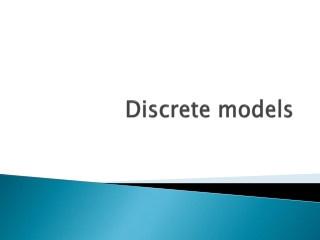DownloadDownload PresentationDiscrete models

Discrete models

Download PresentationDiscrete models

- - - - - - - - - - - - - - - - - - - - - - - - - - - E N D - - - - - - - - - - - - - - - - - - - - - - - - - - -
Presentation Transcript

1. Discrete models

2. Types of discrete models • Binary models Logit and Probit • Binary models with correlation (multivariate) • Multinomial non ordered • Ordered models (rankings) • Count models (patents)

3. We focus on binarymodels • Refer to Greene chapter (also uploaded in the site) and Montini document on fit measures. • Microeconometrics • Consumer choices (but not only) • Random utility framework (linked to Hicksian theory).. You observe what people choose, they choose what the like the best

4. Cdf= cumulative density function

5. Mean and variance for Bernoulli random var • In deciding on the estimation technique, it is useful to derive the conditional mean and variance • E(y/x)= B0 + B1x1 + B2x2 +……Bkxk • Var (y/x)= XB(1- XB), where XB is B0 + B1x1 + B2x2 +……Bkxk. • OLS produces consistent and even unbiased estimates, BUT… • Heteroskedasticity is always an issue to be dealt by weighted least squares (het in stata)

6. HET • Always recall that HET affects s.e. not size of coefficients.. Correction should improve T ratios since it lower variances

7. Linear probability model for binarymodels • P(y=1/X)= B0 + B1x1 + B2x2 +……Bkxk • B1= dP(y=1)/dx1, assuming x1 is not related functionally to other covariates, B1 is the change in the probability of success given a one unit increase in x1. holding other Xj fixed • Unless x is restricted, the LPM cannot be a good description of the population response probability • There are values of Bx for which P is outside the unit 0-1 interval

8. LPM thennotusable • So what? We ve to find a model coherent with a probability framework • Here LOGIT and PROBIT enters

9. Used in MNL contexts

10. The sign is given by the sign of B See Mancinelli, Mazzanti, Ponti and Rizza (2010), J of socio economics, also WP DEIT Non siamo in un contesto dove possiamo rappresentare B come elasticità, questo è vero anche in modelli lin-log, dove ad esempio la var dipendente (causa ‘0’ diffusi) non può essere rappresentata in log.

11. Elasticity • Linear model • Dy/dx = b; e=b*x/y • Log log • Dlny/dlnx= b*y/x • E=b • Lin log • Dy/dlnx = b*1/x • E= b*x/y= b/y

12. See various examples of papers in the site, mainly on innovation variables of adoption that take values 1/0

13. Practical issues to interpretestimates • Coefficient fo not represent marginal effects • You can use dprobit in STATA for that • R2 is not a measure of fit, we have pseudo R2, es. McFadden R2 (see Montini document on that) • You should have good F test, reasonable R2 (0.2 excellent, but 0.05 fine as well), a set of *** coefficients.

14. Goodness of fit See Montini chapter

15. Two stage Heckman • Es. R&D, labor hours offered • First stage probit, then OLS • Get the inverse Mills ratio from first to inform the second and see whether the bias is there • Heckman vs Tobit models (different assumptions)

16. 2 stage Heckman or heckit model: sample selection • Y1= X1B1 + u1 • Y2= 1(x2 + v2 >0) • Hp: x,y2 always observed, y1 only if y2=1, set to 0 if y2=0 • E(y1/X, y2=1) = x1B1 + (x2) • OLS can produce biased inconsistent estimates of B1 if we do not account for the last term • OMITTED VAR problem

17. We need an estimator of 2! • Obtain the probit estimates of 2 from first stage P(y2=1/x) = (x 2) using all N units • Then estimate Inverse Mills ratio,  = f(x2) • Insert IMR in the OLS second equation and get B and  estimates • These estimators are consistent

18. X1 covariates of OLS, X of probit • ** we dont need x1 to be a subset of X for identification. But X=X1 can introduce collinearity since  can be approximated by a linear function of X • Example in Wooldridge, Econometric analysis of cross section and panel data, p.565 (wage equation for married women) • Estimates become imprecise when X=x1